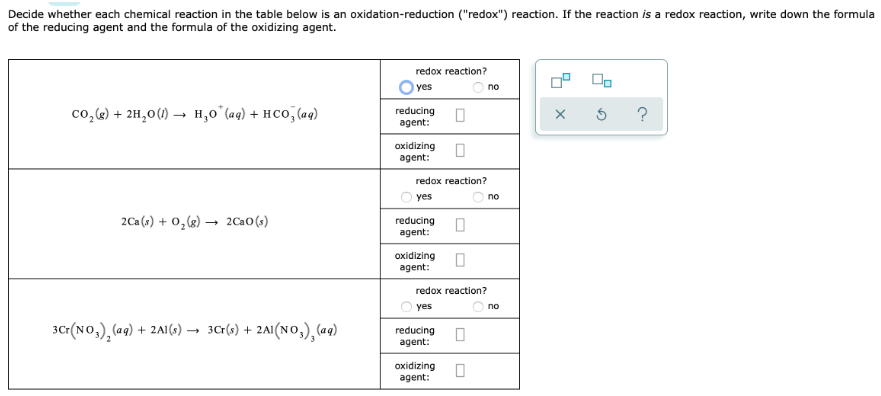# Decide whether each chemical reaction in the table below is an oxidation-reduction ("redox") reaction. If the reaction is a redox reaction, write down the formula of the reducing agent and the formula of the oxidizing agent. a. CO2(g) + 2H2O(l) → H3O+(aq) + HCO3-(aq) b. 2Ca(s) + O2(g) → 2CaO(s) c. 3Cr(NO3)2(aq) + 2Al(s) → 3Cr(s) + 2Al(NO3)3(aq)